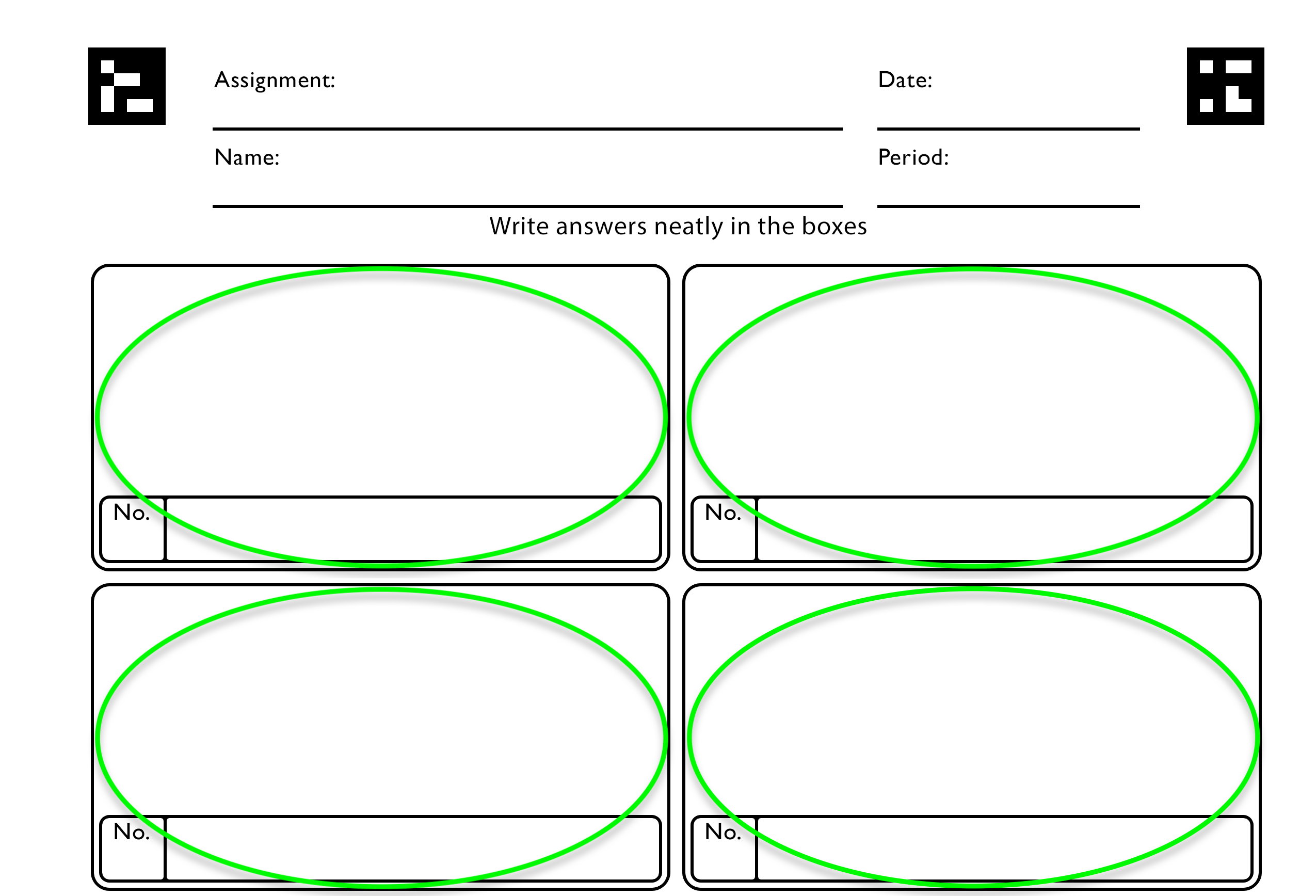# What types of questions can I put into Bakpax?

Currently, Bakpax supports three types of answer submissions. The first two types can be graded by the AI and the third type must be graded by the teacher.

1. Text: Any solution which has alphanumeric characters. Examples of problems which could be graded as text answers are multiple choice (e.g., a, b, c, and d) or short answers that are up to 4 words long (e.g., "apples", "10 apples", and "there were 10 apples").
• Limitation: Longer responses are more difficult to grade as a text answer. The Manual type of solution should be used for long responses. For Text answers, the AI matches submission to an answer which the teacher enters and only marks answers as correct if it matches exactly.
2. Math: Any solution which includes mathematical expressions. Bakpax can recognize fractions, exponents, integrals, and more types of mathematical phrasing.
3. Manual: Any solution which the student draws or writes. When a solution is manual, the student has the entire question/answer box to write in their response. Bakpax then shows the teacher just the question/answer box so that they can easily grade the student response.